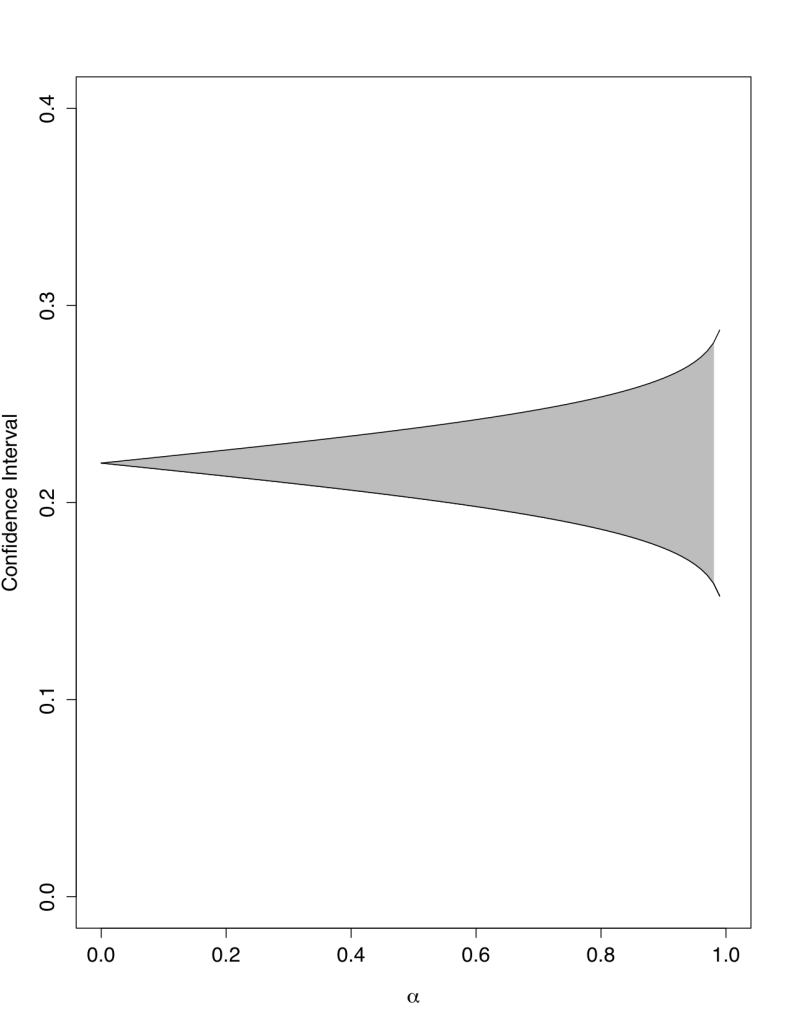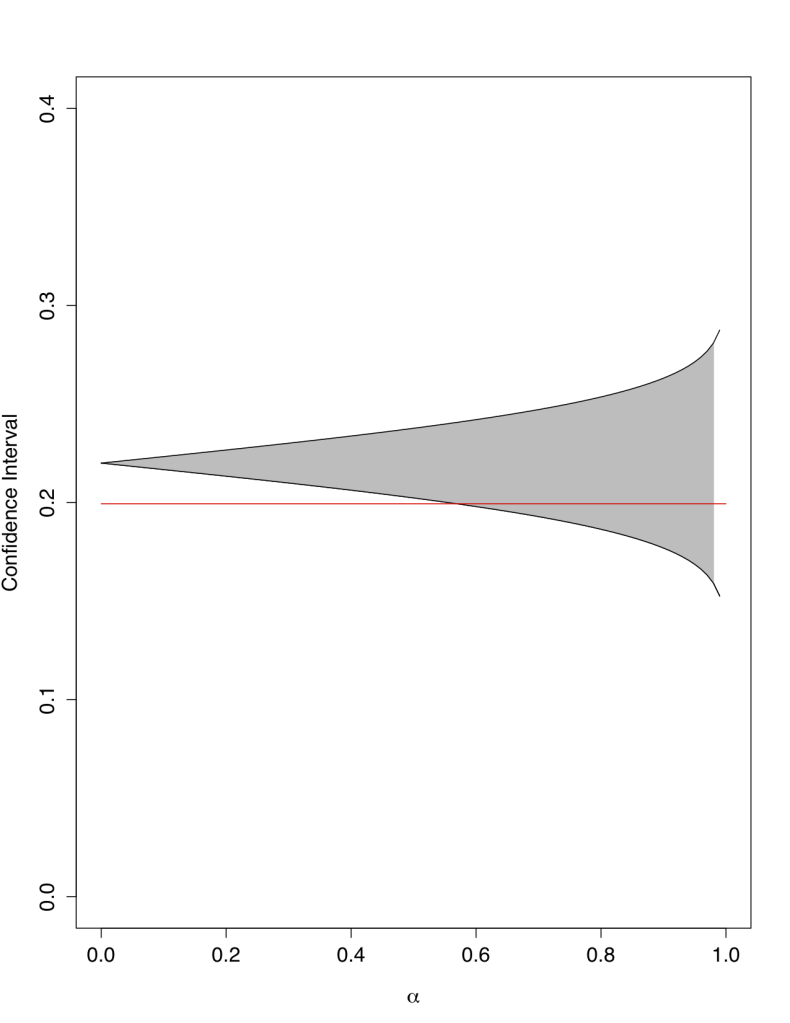[Note: While many of my posts appeal only to readers with certain interests--specifically, mine--this one is meant to provide a public good in the form of an R script that can be run to find multiple confidence intervals around the same sample value. This and other methodological posts in the future may not appeal to the general reader, so they are posted here under the category "Technical." Read only the "Uncategorized" posts if you prefer my random miscellany. Comments from readers of all methodological traditions and experience levels are invited.]

Purpose: Find a series of lower and higher bounds for the confidence interval around a sample statistic.

Script:

###################################################
# Computing a Series of Confidence Levels in R
# Matt Dickenson
# yspr dot wordpress dot com
# Released under a Creative Commons Licence
###################################################

# INSTRUCTIONS
# First, you will need your desired confidence levels, sample statistic, and standard error of the sample statistic
# Compute those, and then run this script
CONFIDENCE <- function(x,y,se){
intervals <- matrix(NA,nrow=(length(x)),ncol=2)
levels <- matrix(rep(x,2),nrow=(length(x)),ncol=2)
rnames <- c()

for (i in 1:(length(x)*2)){
if(i %% 2 != 0){
zi <- qnorm((1-(levels[(floor(i/2)+1),1]))/2)
low <- y+(zi*se)
intervals[floor(i/2)+1,1] <- low
}
else{
zi <- qnorm((1-(levels[(i/2),2]))/2)
high <- y-(zi*se)
intervals[(i/2),2] <- high

}
}
row.names(intervals) <- x
colnames(intervals) <-c("Lower Bound", "Higher Bound")
intervals
}
# Now, run the command as
CONFIDENCE(x,y,se)
# CONDITIONS:
# where x is the vector containing your desired confidence levels (0<x[i]<1 for all i)
# and y is your sample statistic and se is the standard error of your sample statistic
# note that the actual variable names can be anything you want, as long as they are entered in this order

Notice that in less than twenty actual lines of code we have developed a function that can find any number of normal distribution confidence intervals. It is also flexible, and with just a bit of tweaking you could change this to another distribution, like Student's t, binomial, or Poisson. Here is an example of one use for this function:

# EXAMPLE
# Finding the porportion of mortgage holders who had subprime mortgages
ptrue <- mean(subprime\$high.rate, na.rm=T)

# Drawing a simple random sample of the population
set.seed(126)
phat <- mean(subprime.sample, na.rm=T)
phat
# =0.22
# computing standard error of sample mean
sampleSE <- sqrt((phat*(1-phat)/length(subprime.sample)))

# Say we want to find the 50, 95, and 99 percent confidence interval estimates...
conlev <- c(.50,.95,.99)
CONFIDENCE(conlev, phat, sampleSE)
> CONFIDENCE(conlev, phat, sampleSE)
Lower Bound Higher Bound
0.5    0.2023289    0.2376711
0.95   0.1686504    0.2713496
0.99   0.1525152    0.2874848
# What about including the ninety percent confidence intervals?
conlev2 <-c(.50, .90, .95, .99)
CONFIDENCE(conlev2, phat, sampleSE)
> CONFIDENCE(conlev2, phat, sampleSE)
Lower Bound Higher Bound
0.5    0.2023289    0.2376711
0.9    0.1769061    0.2630939
0.95   0.1686504    0.2713496
0.99   0.1525152    0.2874848
# How about an interval for each percentile?
CONFIDENCE(seq(0,1,by=.01))
# output not shown for the sake of space, BUT
# We can graph these:
x <- seq(0,1,by=.01)
y <- CONFIDENCE(x,phat,sampleSE)
library(grDevices)
plot(x,y[,1], type="l", ylim=c(0,0.4), xlim=c(0,1), ylab="Confidence Interval", xlab=expression(alpha))
polygon(c(x,x[2:98],x,x,x[98:2],x), c(y[1,2],y[2:98,2],y[99,2],y[99,1],y[98:2,1],y[1,1]), border="grey",col="grey")
lines(x,y[,1], type="l")
lines(x,y[,2], type="l")

Here is the plot:The size of the confidence level (the grey area) increases as we seek more confidence about our estimate. Since we set the seed to 126, your random sample should be exactly the same as mine, which allows for direct comparison of results for the example.

# How does this compare to the true population proportion?
points(x,y=(rep(ptrue, length(x))),type="l", col="red")

Here is the new plot:We can see that we have included the true population proportion by the time our confidence level grows to include 60 percent of the distribution of our sample proportion.

Feedback is welcome in the comments, especially links to scripts that modify this function.